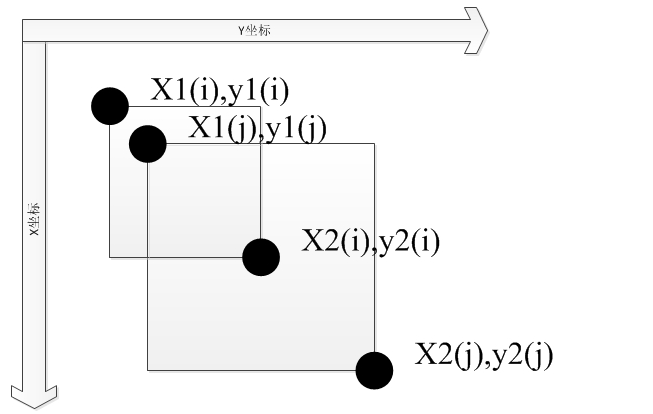# 非最大抑制（NMS）

<span style="font-family: 'Microsoft YaHei';">转之：</span>

http://blog.csdn.net/H2008066215019910120/article/details/25917609#

非极大值抑制顾名思义就是抑制不是极大值的元素，搜索局部的极大值。这个局部代表的是一个邻域，邻域有两个参数可变，一是邻域的维数，二是邻域的大小。这里不讨论通用的NMS算法，而是用于在目标检测中用于提取分数最高的窗口的。例如在行人检测中，滑动窗口经提取特征，经分类器分类识别后，每个窗口都会得到一个分数。但是滑动窗口会导致很多窗口与其他窗口存在包含或者大部分交叉的情况。这时就需要用到NMS来选取那些邻域里分数最高（是行人的概率最大），并且抑制那些分数低的窗口。

function pick = nms(boxes, overlap)

% pick = nms(boxes,overlap)
% Non-maximumsuppression.
% Greedily selecthigh-scoring detections and skip detections
% that are significantlycovered by a previously selected detection.

% boxes = boxes';

if isempty(boxes)
pick = [];
else
x1 = boxes(:,1);
y1 = boxes(:,2);
x2 = boxes(:,3);
y2 = boxes(:,4);
%     x1 = boxes(:,1);
%     y1 = boxes(:,2);
%     x2 = boxes(:,2);
%     y2 = boxes(:,4);
s = boxes(:,end);
area = (x2-x1+1) .* (y2-y1+1);%Çó³öËùÓÐÃæ»ý

[vals, I] = sort(s);
pick = [];
while ~isempty(I)
last = length(I);
i = I(last);
pick = [pick; i];
suppress = [last];
for pos = 1:last-1
j = I(pos);
xx1 = max(x1(i), x1(j));
yy1 = max(y1(i), y1(j));
xx2 = min(x2(i), x2(j));
yy2 = min(y2(i), y2(j));
w = xx2-xx1+1;
h = yy2-yy1+1;
if w > 0 && h > 0
% compute overlap
o = w * h / min(area(i),area(j));
%                 o = w/area(j);
if o > overlap
suppress = [suppress; pos];
end
end
end
I(suppress) = [];
end
end


输入的窗口的位置和分数，以及窗口面积交叉是多大比例进行抑制。boxes应当是N*5的矩阵，一行代表着一个窗口，包括[x,y,width,height ,score ]，overlap是介于0~1的实数。输出的是局部分数最大的窗口序号序列。

程序解读：首先计算出所有窗口的面积，对所有窗口的分数进行从小到大排序取出最高分数的序号。循环计算1到次高分数窗口与最高分数窗口的交叉面积与两者间最小面积的比例，若超过overlap那么把这一窗口(1~~last中的窗口)抑制了。交叉面积怎么计算呢？如下图对应于程序

            xx1 = max(x1(i), x1(j));
yy1 = max(y1(i), y1(j));
xx2 = min(x2(i), x2(j));
yy2 = min(y2(i), y2(j));
w = xx2-xx1+1;
h = yy2-yy1+1;
if w > 0 && h > 0
% compute overlap
o = w * h / min(area(i),area(j));
if o > overlap
suppress = [suppress; pos];
end
endhttp://www.pyimagesearch.com/2015/02/16/faster-non-maximum-suppression-python

# import the necessary packages
import numpy as np

# Malisiewicz et al.
def non_max_suppression_fast(boxes, overlapThresh):
# if there are no boxes, return an empty list
if len(boxes) == 0:
return []

# if the bounding boxes integers, convert them to floats --
# this is important since we'll be doing a bunch of divisions
if boxes.dtype.kind == "i":
boxes = boxes.astype("float")

# initialize the list of picked indexes
pick = []

# grab the coordinates of the bounding boxes
x1 = boxes[:,0]
y1 = boxes[:,1]
x2 = boxes[:,2]
y2 = boxes[:,3]

# compute the area of the bounding boxes and sort the bounding
# boxes by the bottom-right y-coordinate of the bounding box
area = (x2 - x1 + 1) * (y2 - y1 + 1)
idxs = np.argsort(y2)

# keep looping while some indexes still remain in the indexes
# list
while len(idxs) > 0:
# grab the last index in the indexes list and add the
# index value to the list of picked indexes
last = len(idxs) - 1
i = idxs[last]
pick.append(i)

# find the largest (x, y) coordinates for the start of
# the bounding box and the smallest (x, y) coordinates
# for the end of the bounding box
xx1 = np.maximum(x1[i], x1[idxs[:last]])
yy1 = np.maximum(y1[i], y1[idxs[:last]])
xx2 = np.minimum(x2[i], x2[idxs[:last]])
yy2 = np.minimum(y2[i], y2[idxs[:last]])

# compute the width and height of the bounding box
w = np.maximum(0, xx2 - xx1 + 1)
h = np.maximum(0, yy2 - yy1 + 1)

# compute the ratio of overlap
overlap = (w * h) / area[idxs[:last]]

# delete all indexes from the index list that have
idxs = np.delete(idxs, np.concatenate(([last],
np.where(overlap > overlapThresh))))

# return only the bounding boxes that were picked using the
# integer data type
return boxes[pick].astype("int")